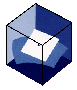This site is based on the article V.L. Hansen: I am the Greatest, Mathematics in School Vol.25, No.4, 1996, 10-11.Curriculum VitaeRPAMath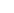Mathematics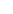I am the greatestPostScriptAcrobat Reader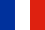Web I AM THE GREATEST Vagn Lundsgaard Hansen Optimality considerations enter into almost all physical and technological design problems. Optimal design of structures (girders, sheets etc) utilising in the most efficient way the basic properties of materials, is a very important research area in modern technology, yet optimality questions are rarely raised in lower grades as mathematical questions. In this article I shall tell the story of the quadrilaterals with fixed perimeter which competed for enclosing the largest area. The story is an introduction to the isoperimetric problem and can be presented at a relatively early level in the school system. It can easily be arranged as a short play with students playing the roles of various quadrilaterals. Setting up a competition In the world of mathematics once it was announced that there should be a competition among all quadrilaterals to see which one enclosed the largest area. To make the competition fair the quadrilaterals competed in different classes so that only quadrilaterals with the same perimeter should be compared. In the announcement it said that isoperimetric quadrilaterals should be examined to find out which one enclosed the largest area. Scholars knew that `iso' is the Greek word for `same' and that `perimeter' derives from the Greek words `peri' meaning `around' and `metron' meaning `measure', and accordingly that the perimeter of a quadrilateral is the sum of the lengths of the sides. They further knew that mathematics owes much to the Greeks. The text of the announcement was in fact formulated as a mathematical problem. Problem: Among all quadrilaterals with a prescribed perimeter find the one enclosing the largest area. All the quadrilaterals looked forward to the day of the competition. Everyone thought that it would be the winner. Some had a re-entrant angle, some were parallelograms, others were rectangles and many had sides of different lengths. In the background you saw the square. The competition Finally the day for the competition arrived. The referee of the competition called upon all the quadrilaterals with the prescribed perimeter L. A quadrilateral with perimeter L having a re-entrant angle as in Figure 1 stepped forward, presenting itself as Mr. Concave and saying: "I enclose the largest area." The referee smiled and said: "No, Mr. Concave, this cannot be true since by reflecting your inbuckling into an outbuckling, I can create a quadrilateral with a larger area than you but still with perimeter L." The referee actually made a figure (Figure 2) so that Mr. Concave could see it for himself.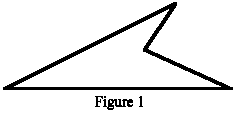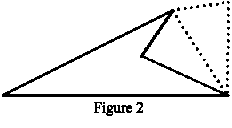The referee told Mr. Concave that since it was not a convex quadrilateral, i.e. it did not contain both of its diagonals, it could not hope for enclosing the largest area. Consequently, the referee asked all quadrilaterals, which were not convex, to step down since none of them had any chance of enclosing the largest area. Back in the competion now remained all convex quadrilaterals with the perimeter L. Then Mr. Uneven (Figure 3), which had adjacent sides of different lengths, stepped forward and proclaimed that it was the quadrilateral enclosing the largest area. The referee took a short look at it and then suddenly divided it by a diagonal as in Figure 4. Mr. Uneven got confused and very disappointed when the referee said: "No, Mr. Uneven, you are not the quadrilateral enclosing the largest area, since by replacing your adjacent uneven sides by sides of the same length, I can create a quadrilateral enclosing a larger area than you and still with perimeter L." Mr. Uneven did not quite understand it, but the referee told him to go home and convince himself that a triangle with a fixed base and the sum of the two other sides a fixed constant encloses the largest area when it is an isosceles triangle over the base. The referee further told Mr. Uneven that he could figure that out by observing that the corner opposite the base in such a triangle must lie on an ellipse with the endpoints of the base as foci and consequently, that the height on the base is greatest possible exactly when the triangle is isosceles. Mr. Uneven had to do some studies before he was convinced, but eventually he was.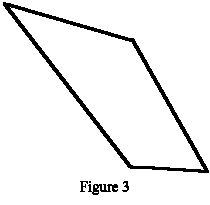After the incident with Mr. Uneven, the referee concluded, that to find out which quadrilateral encloses the largest area, he only needed to consider those convex quadrilaterals as in Figure 5, which by a diagonal could be split into two isosceles triangles. Back in the competition therefore now only remained such quadrilaterals with perimeter L.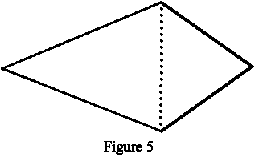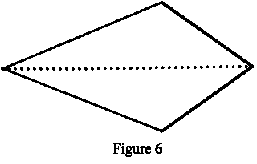Mr. Kite eagerly pushed on. He was a quadrilateral as in Figure 5 with perimeter L, which by a diagonal could be split into two isosceles triangles with the two pairs of legs of different lengths. Mr. Kite said: "Now that all the others have been sent away, surely I must be the quadrilateral enclosing the largest area." The referee took a close look at Mr. Kite. Then suddenly he said "ahah" and divided it by the other diagonal as shown in Figure 6. Mr. Kite got a chock and while he was recovering, he heard the referee saying that Mr. Kite did not enclose the largest area after all since one could make a quadrilateral with an even larger area by replacing the two symmetric triangles with the diagonal as base by isosceles triangles. Realising that this was true, Mr. Kite and all his peers left the competition. Back in the competition now were the quadrilaterals having all the sides of equal length. Mr. Rhombus (Figure 7) stepped forward and said: "It is rather easy to compute the area of me, it is just the product of my height h and the length of my side." "Yes", said the referee, "this is right, and do you then know who is the winner?" After a short while, Mr. Rhombus said: "Since my area is largest when my height h is largest, I enclose the largest area when my angles are right angles." "Absolutely correct", said the referee, "and what you get is a square (Figure 8)."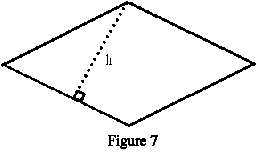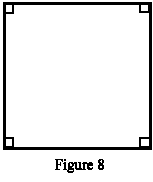The referee then took the microphone and pronounced: Among all quadrilaterals with a prescribed perimeter, the square encloses the largest area. The competition was over. The quadrilaterals went home. The isoperimetric problem for quadrilaterals had been solved. The square was the winner. And so it has always been and so it will always be, but now it had been settled beyond any doubts. In mathematics they call this fact about the square a theorem and the explanation given for it a proof. The isoperimetric problem for closed polygons and curves Somehow the isoperimetric problem is slightly more difficult for triangles than for quadrilaterals. The problem can be formulated as follows: Among all triangles with a prescribed perimeter find the one that encloses the largest area. The problem can be tackled as follows. Start out with an arbitrary triangle with prescribed perimeter L. By an iterative process, we can construct a sequence of triangles with perimeter L with increasing area by making the triangle isosceles over each one of the sides in turn. This (infinite) sequence of triangles in the limit approaches the equilateral triangle with perimeter L. By continuity of the area of a triangle on its shape (Heron's formula) it follows that the equilateral triangle is the triangle enclosing the largest area among all triangles with the prescribed perimeter L. The isoperimetric problem for general n-gons needs a little more machinery; cf. [Hansen, 1992]. But the result is as one would expect by now, namely: Theorem 1: Among all closed polygons with n sides and with a prescribed perimeter, the regular n-gon encloses the largest area. For closed curves in the plane without self-intersections and with finite length (rectifiable Jordan curves) one has the general isoperimetric problem. In this case, the circle is the solution. Theorem 2: Among all closed curves in the plane without self-intersections and with a prescribed length (rectifiable Jordan curves), the circle encloses the largest area. In [Hansen, 1994] a physical experiment is described by which you can demonstrate that the circle encloses the largest area compared to its perimeter. In the references below you can find other optimization problems from geometry with relation to real world phenomena. Litterature: Eggleston, H.G. (1967), The Isoperimetric Problem, Chapter 7, in "Exploring University Mathematics 1" (N.J. Hardiman, ed.), Pergamon Press, 1967. Hansen, V.L. (1992), "Temaer fra Geometrien," Matematiklærerforeningen, 1992. See also: "Shadows of the Circle," World Scientific, Singapore, 1998. Hansen, V.L. (1993), "Geometry in Nature," A K Peters, Ltd., Wellesley, Massachusetts, US, 1993. Hansen, V.L. (1994), The Magic World of Geometry -I. The Isoperimetric Problem, Elemente der Mathematik Vol. 49, No. 2 (1994), pp. 61--65. Hildebrandt, S. and Tromba, A. (1985), "Mathematics and Optimal Form," Scientific Amer. Library, W.H. Freeman and Co, 1985. Kline, M. (1972), "Mathematical Thought from Ancient to Modern Times," Oxford University Press, 1972. A Danish translation: Jeg er den største, has been published in Nordisk Matematisk Tidskrift Vol.46, No.2, 1998, 71-75. Available as:PostScriptpdf A French translation: Un conte de fées mathématique, has been published in the booklet Mathématiques Buissonnières en Europe, pages 11-13, published by Editions Vuibert for the French Ministère de l'Education Nationale et Ministère de la Recherche, October 2000. Available as: pdf The French translation is also available in the electronic library of the Association pour le Développement de la Culture Scientifique: Web